# NCERT Solutions class 7 Maths Chapter-15 Exercise 15.1

## NCERT Solutions class 7 Maths Chapter 15 Visualising Solid Shapes

### Symmetry Exercise-15.1

NCERT Solutions Class-7 Maths chapter 15 Visualising Solid Shapes Exercise-15.1 is prepared by academic team of Physics Wallah all the questions of NCERT text book are solved step by step with proper and detail solutions explaining each and every questions.For More and additional questions of CBSE class 7 maths you can go to class 7 maths sections. NCERT class 7 Maths Solutions is the best way to enhanced your mathematics skill. And Physics Wallah practice worksheet & question bank will help you a lot .

### Solutions of Chapter Visualising Solid Shapes Exercise-15.1

Q 1. Identify the nets which can be used to make cubes (cut out copies of the nets and try it)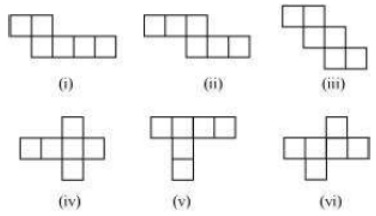Ans:

(i) The given net can be folded as follows.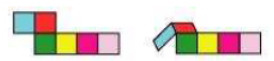When the faces that are in sky blue colour and in pink colour are folded to make a cube, they will be overlapping each other.

(ii) The given net can be folded as follows.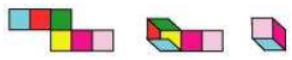A cube can thus be formed in the above way.

(iii) The given net can be folded as follows.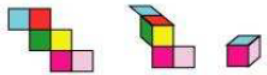A cube can thus be formed in the above way.

(iv) The given net can be folded as follows.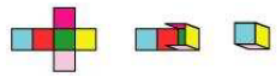A cube can thus be formed in the above way.

(v) The given net can be folded as follows.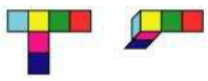When the faces that are in blue colour and in red colour are folded to make a cube, they will be overlapping each other.

(vi) The given net can be folded as follows.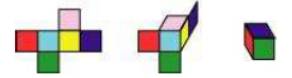A cube can thus be formed in the above way.

Q 2. Dice are cubes with dots on each face. Opposite faces of a die always have a total of seven dots on them.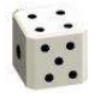Here are two nets to make dice (cubes); the numbers inserted in each square indicate the number of dots in that box.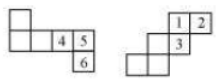Insert suitable numbers in the blanks, remembering that the number on the opposite faces should total to 7.

Ans:

(i) The numbers can be inserted as follows so as to make the given net into a net of a dice.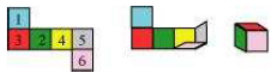It can be observed that the sum of the opposite faces is 7.

(ii)The numbers can be inserted as follows so as to make the given net into a net of a dice.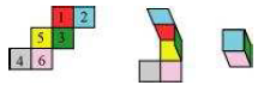It can be observed that the sum of the opposite faces is 7.

Q 3. Can this be a net for a die?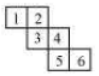Ans: The given net can be folded as follows.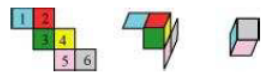It can be observed that the opposite faces of the dice so formed have 2 and 5, 1 and 4, 3 and 6 on them. The sum of the numbers on the opposite faces comes to 7, 5, 9 respectively. However, in case of a dice, the sum of the numbers on the opposite faces should be 7. Hence, this net is not of a dice.

Q 4.  Here is an incomplete net for making a cube. Complete it in at least two different ways. Remember that a cube has six faces. How many are there in the net here? (Give two separate diagrams. If you like, you may use a squared sheet for easy manipulation.)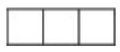Ans: There are 3 faces in the given net. The given net can be completed as follows.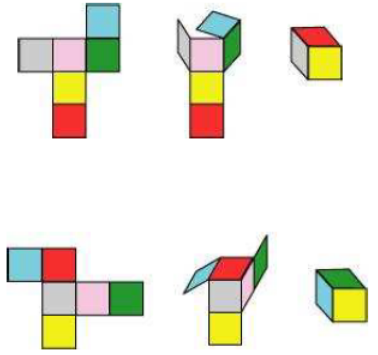Q 5. Match the nets with appropriate solids: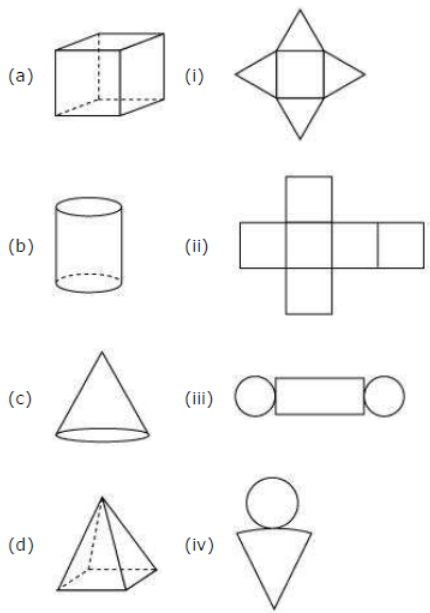Ans:

Consider net (i). It can be folded as follows.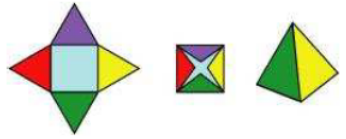It is a net of a pyramid. Hence, (d) is the correct matching option.

Consider net (ii). It can be folded as follows.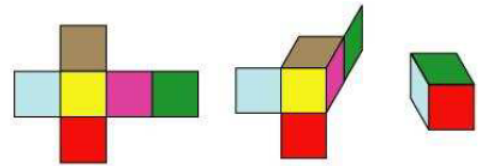It is a net of a cube. Hence, (a) is the correct matching option.

Consider net (iii). It can be folded as follows.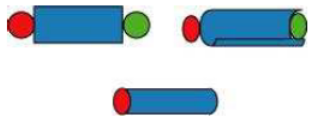It is a net of a cylinder. Hence, (b) is the correct matching option.

Consider net (iv). It can be folded as follows.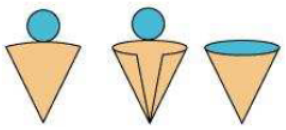It is a net of a cone. Hence, (c) is the correct matching option.

NCERT CLASS 7 MATHEMATICS SOLUTIONS